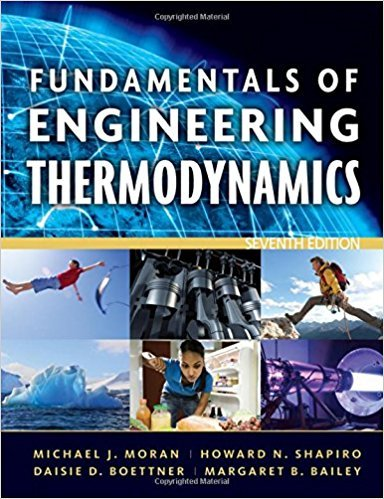×
Get Full Access to Fundamentals Of Engineering Thermodynamics - 7 Edition - Chapter 13 - Problem 112p
Get Full Access to Fundamentals Of Engineering Thermodynamics - 7 Edition - Chapter 13 - Problem 112p

×

# Exergy Analysis of Reacting and Psychrometric | Ch 13 - 112PISBN: 9780470495902 50

## Solution for problem 112P Chapter 13

Fundamentals of Engineering Thermodynamics | 7th Edition

• Textbook Solutions
• 2901 Step-by-step solutions solved by professors and subject experts
• Get 24/7 help from StudySoup virtual teaching assistantsFundamentals of Engineering Thermodynamics | 7th Edition

4 5 1 394 Reviews
28
2
Problem 112P

PROBLEM 112P

Exergy Analysis of Reacting and Psychrometric Systems

For each of the following, use the result of Problem 13.111(a) to determine the total specific flow exergy, in kJ/kg, relative to an environment consisting of moist air at 20°C, 1 atm, ϕ = 100%

(a) moist air at 20°C, 1 atm, ϕ = 90%.

(b) moist air at 20°C, 1 atm, ϕ = 50%.

(c) moist air at 208C, 1 atm, ϕ = 10%.

Problem 13.111(a)

Exergy Analysis of Reacting and Psychrometric Systems

For psychrometric applications such as those considered in Chap. 12, the environment often can be modeled simply as an ideal gas mixture of water vapor and dry air at temperature T0 and pressure p0. The composition of the environment is defined by the dry air and water vapor mole fractions,, respectively.

(a) Show that relative to such an environment the total specific flow exergy of a moist air stream at temperature T and pressure p with dry air and water vapor mole fractions ya and yv, respectively, can be expressed on a molar basis asStep-by-Step Solution:
Step 1 of 3

The Future Climate Introduction:  The IPCC stated in 2013: "Warming of the climate system is unequivocal, and since the 1950s, many of the observed changes are unprecedented over decades to millennia." So what will happen in the future  Predicting the future for our climate is more difficult to guess  Knowing the composition of the atmosphere, the solar constant, the albedo of the earth helps understand what the future temperature will be There are three key factors that control greenhouse gas emissions 1 Population 1 Affluence (How rich individual people are) 1 Technology  Together, these make up the formula I=PAT , which can be used to predict the impacts of energy consumption o (Impact = Population x Aff

Step 2 of 3

Step 3 of 3

##### ISBN: 9780470495902

The full step-by-step solution to problem: 112P from chapter: 13 was answered by , our top Engineering and Tech solution expert on 07/20/17, 09:01AM. Since the solution to 112P from 13 chapter was answered, more than 282 students have viewed the full step-by-step answer. Fundamentals of Engineering Thermodynamics was written by and is associated to the ISBN: 9780470495902. The answer to “Exergy Analysis of Reacting and Psychrometric SystemsFor each of the following, use the result of 13.111(a) to determine the total specific flow exergy, in kJ/kg, relative to an environment consisting of moist air at 20°C, 1 atm, ? = 100%(a) moist air at 20°C, 1 atm, ? = 90%.________________(b) moist air at 20°C, 1 atm, ? = 50%.________________(c) moist air at 208C, 1 atm, ? = 10%. 13.111(a)Exergy Analysis of Reacting and Psychrometric SystemsFor psychrometric applications such as those considered in Chap. 12, the environment often can be modeled simply as an ideal gas mixture of water vapor and dry air at temperature T0 and pressure p0. The composition of the environment is defined by the dry air and water vapor mole fractions , , respectively. (a) Show that relative to such an environment the total specific flow exergy of a moist air stream at temperature T and pressure p with dry air and water vapor mole fractions ya and yv, respectively, can be expressed on a molar basis as” is broken down into a number of easy to follow steps, and 170 words. This textbook survival guide was created for the textbook: Fundamentals of Engineering Thermodynamics, edition: 7. This full solution covers the following key subjects: air, moist, Environment, atm, exergy. This expansive textbook survival guide covers 14 chapters, and 1501 solutions.

Unlock Textbook Solution